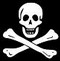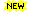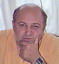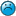GotAI.NET

# Форум: Проблемы искусственного интеллекта

 Все темы | Новая тема Стр.48 (51) << < Пред. | След. > >> Поиск:
Автор Тема: На: False AI. Немодерируемая ветка про ИИ. Без оффтопиков, хамства и болтунов
 гостСообщений: 6163На: False AI. Немодерируемая ветка про ИИ. Без оффтопиков, хамства и болтуновДобавлено: 06 сен 15 7:26
Изменено: 07 сен 15 1:55
,

,
,
,
,

,
,

Find the difference directly from

,

,

This is the correct formula but it is necessary to pay attention:

The metric is different for and , and have a difference in dimensional scale and total value in the general.
 [Ответ][Цитата]
 гостСообщений: 6163На: False AI. Немодерируемая ветка про ИИ. Без оффтопиков, хамства и болтуновДобавлено: 07 сен 15 4:36
Изменено: 08 сен 15 4:52
Model of continuum

Vortex lines in the continuum - line direction instantaneous axis of rotation of the particles are on them.
Vortex filaments in a continuum - Dedicated mass, each element surface boundary set according vortex line .

Suppose that for all the forces acting on the environment there is a potential strength.
We define potential 0-forms:

Kinematic viscosity or Magnetic weight is 0-form of primal nodes.
Thin magnetic solenoid - thread magnetized liquid crystal, which can be represented as a line current I is 0-form of dual nodes.

Faraday's law of induction makes use of the magnetic flux through a hypothetical surface whose boundary is a wire loop. Since the wire loop may be moving, we write for the surface. The magnetic flux is defined by a surface integral:

,

The product of the cross section of the speed of rotation for an infinitely thin filament (2 form) to all over her constantly and stores the value in the movement of the thread .
 [Ответ][Цитата]
 NОСообщений: 77На: False AI. Немодерируемая ветка про ИИ. Без оффтопиков, хамства и болтуновДобавлено: 07 сен 15 5:01
 Цитата:
 Автор: ignat99, ,,,,,,Find the difference directly from ,,This is the correct formula but it is necessary to pay attention:The metric is different for and , and have a difference in dimensional scale and total value in the general.
выглядит зловеще
 [Ответ][Цитата]
 гостСообщений: 6163На: False AI. Немодерируемая ветка про ИИ. Без оффтопиков, хамства и болтуновДобавлено: 07 сен 15 5:08
Изменено: 07 сен 15 5:45
 Цитата:
 Автор: Владимир Пахомоввыглядит зловеще

Model of continuum

Vortex lines in the continuum - line direction instantaneous axis of rotation of the particles are on them.
Vortex filaments in a continuum - Dedicated mass, each element surface boundary set according vortex line .

Suppose that for all the forces acting on the environment there is a potential strength.
We define potential 0-forms:

Kinematic viscosity or Magnetic weight is 0-form of primal nodes.
Thin magnetic solenoid - thread magnetized liquid crystal, which can be represented as a line current I is 0-form of dual nodes.

Faraday's law of induction makes use of the magnetic flux through a hypothetical surface whose boundary is a wire loop. Since the wire loop may be moving, we write for the surface. The magnetic flux is defined by a surface integral:

is primal node (0-form),

where dA is an element of surface area of the moving surface , B is the magnetic field (also called "magnetic flux density"), and B·dA is a vector dot product (the infinitesimal amount of magnetic flux through the infinitesimal area element dA). In more visual terms, the magnetic flux through the wire loop is proportional to the number of magnetic flux lines that pass through the loop.

The product of the cross section of the speed of rotation for an infinitely thin filament (2-form) to all over her constantly and stores the value in the movement of the thread . In other words, the magnetic mass of filaments remain unchanged during the motion.

Or for NS:

is primal edges (1-form),
is primal 2-cell,

Vortex filaments it is dual node (not real vector):

is dual 0-form.
 [Ответ][Цитата]
 NОСообщений: 77На: False AI. Немодерируемая ветка про ИИ. Без оффтопиков, хамства и болтуновДобавлено: 07 сен 15 5:41

 [Ответ][Цитата]
 гостСообщений: 6163На: False AI. Немодерируемая ветка про ИИ. Без оффтопиков, хамства и болтуновДобавлено: 07 сен 15 5:47
Изменено: 07 сен 15 15:03
Model of continuum

Vortex lines in the continuum - line direction instantaneous axis of rotation of the particles are on them.
Vortex filaments in a continuum - Dedicated mass, each element surface boundary set according vortex line .

Suppose that for all the forces acting on the environment there is a potential strength.
We define potential 0-forms:

Kinematic viscosity or Magnetic weight is 0-form of primal nodes.
Thin magnetic solenoid - thread magnetized liquid crystal, which can be represented as a line current I is 0-form of dual nodes.

Faraday's law of induction makes use of the magnetic flux through a hypothetical surface whose boundary is a wire loop. Since the wire loop may be moving, we write for the surface. The magnetic flux is defined by a surface integral:

is primal node (0-form),

where dA is an element of surface area of the moving surface , B is the magnetic field (also called "magnetic flux density"), and B·dA is a vector dot product (the infinitesimal amount of magnetic flux through the infinitesimal area element dA). In more visual terms, the magnetic flux through the wire loop is proportional to the number of magnetic flux lines that pass through the loop.

The product of the cross section of the speed of rotation for an infinitely thin filament (2-form) to all over her constantly and stores the value in the movement of the thread . In other words, the magnetic mass of filaments remain unchanged during the motion.

Or for NS:

is primal edges (1-form),
is primal 2-cell,

Vortex filaments it is dual node (not real vector):

is dual 0-form.Hill's spherical vortex

H is 1-form may be referred to as the normal velocity form of the Vortex filaments in a continuum or the flux, since it represents the velocity normal to the triangles’ faces (i.e. dual edges).

We denote the velocity 1-form defined on the primal edges by v; i.e. . The velocity 1-form v represents the velocity tangential to the triangles edges.

 H.Helmholtz, Zwei hydrodynamische Abhandlungen. Monatsberichte d. konigl. Acad. d. Wiss. zu Berlin, 1868, S. 215-228
 [Ответ][Цитата]
 гость109.62.143.*
На: False AI. Немодерируемая ветка про ИИ. Без оффтопиков, хамства и болтуновДобавлено: 07 сен 15 6:30
Аминь...
 [Ответ][Цитата]
 гостСообщений: 6163На: False AI. Немодерируемая ветка про ИИ. Без оффтопиков, хамства и болтуновДобавлено: 07 сен 15 6:53
Изменено: 07 сен 15 7:08
 [Ответ][Цитата]
 гостСообщений: 6163На: False AI. Немодерируемая ветка про ИИ. Без оффтопиков, хамства и болтуновДобавлено: 07 сен 15 10:47
Изменено: 07 сен 15 15:14
Consider the components (the convective terms) of the equation associated with the longitudinal force stiffness:

~

I gave an algebraic graph:

A->I->H->B->div B,

A->I is the transition from 1-form to 0-form in the one-dimensional space,

H->B is the transition from 1-form to 2-form of 3-dimensional space,

B->div B is primal div.

Each rotating fluid particle A calls in each of the other particles B the same fluid mass speed. The direction perpendicular to the plane passing through the axis of rotation of A and particle B. A particle velocity is proportional to the volume, rate of rotation B, perpendicular to the axis of rotation, and inversely proportional to the square of the distances between the particles.
 [Ответ][Цитата]
 гостСообщений: 6163На: False AI. Немодерируемая ветка про ИИ. Без оффтопиков, хамства и болтуновДобавлено: 07 сен 15 11:14
Изменено: 07 сен 15 15:16
Similarly the viscous terms, for the lateral force rigidity:

~

Diagram:

A->B->H->J->divB

A->B is primal rot
B<-H is the transition from H 1-form to B 2-form in the 3-dimensional space
H->J is dual rot
J<-div B is the transition from J 2-form to div B 3-form of 5-dimensional space,

Any transition possible see on Diagram 5.
http://www.gotai.net/forum/default.aspx?postid=133588#133588

This component provides a seal and bending into the vortex filaments under the influence of viscosity.
 [Ответ][Цитата]
 NеwPоiskСообщений: 174На: False AI. Немодерируемая ветка про ИИ. Без оффтопиков, хамства и болтуновДобавлено: 07 сен 15 14:04
 Цитата:
 Автор: ignat99
Вы образованный, с этим никто не поспорит, но в праздности потеряли чувство меры и пересытили публику наукообразным контентом, непонятно зачем

дайте ссылки на Ваши научные статьи в соответствующих местах, раз такое дело, такие энциклопедисты как Вы оцениваются по количеству и качеству реферальности, нужно чтобы авторитеты Вас лайкнули, предоставьте доказательства
 [Ответ][Цитата]
 гость95.71.120.*
На: False AI. Немодерируемая ветка про ИИ. Без оффтопиков, хамства и болтуновДобавлено: 07 сен 15 23:49
Простите Ньюпор, Вам шашечки или ехать?
 [Ответ][Цитата]
 гость81.7.17.*
На: False AI. Немодерируемая ветка про ИИ. Без оффтопиков, хамства и болтуновДобавлено: 08 сен 15 2:09
 Цитата:
 Автор: гостьПростите Ньюпор, Вам шашечки или ехать?
это не NewPoisk а его клон-прилипала, созданный им для пиара, но это не факт, в общем стоит воспринимать его как отдельную сущность
 [Ответ][Цитата]
 гостСообщений: 6163На: False AI. Немодерируемая ветка про ИИ. Без оффтопиков, хамства и болтуновДобавлено: 08 сен 15 4:04
Изменено: 08 сен 15 8:17
For NS:

is primal edges (1-form),
is primal 2-cell,

Vortex filaments it is dual node (not real vector):

is dual 0-form.

http://geometry.caltech.edu/pubs/DKT05.pdf
4.3.2 Examples - show in detail the chain complex for the spaces of discrete forms and for the coboundary operator is called the discrete deRham complex (or sometimes, the cochain complex).

The paper detailed mechanisms using large sparse matrices to calculate the homology basis and determine the expression for the finite element (such as the ribs and the values at the vertices).

Is possible to use the device matrix to selectively each finite element calculation. For full the chain complex we make the boundary operator and the exterior derivative operator by matrix. Each transformation matrix for each hierarchy in the chains depending on the selected vector chain.

In the direction from the senior to the junior dimensions of the cells it will be the operators of borders.
In the opposite direction of the diagram it the exterior derivative operator.

Notice how the change in applied mathematics, now it includes algorithms for the calculation of large matrices or simplex complexes (essentially brute force).

 [Ответ][Цитата]
 ЭСГТРСообщений: 8449На: False AI. Немодерируемая ветка про ИИ. Без оффтопиков, хамства и болтуновДобавлено: 08 сен 15 6:04
Изменено: 08 сен 15 6:06
 Цитата:
 Автор: ГостьНемодерируемая ветка про ИИ. Без оффтопиков, хамства и болтунов
Это что же получается без Хмура и Валька????[Ответ][Цитата]
 Стр.48 (51): 1  ...  44  45  46  47    49  50  51 << < Пред. | След. > >>